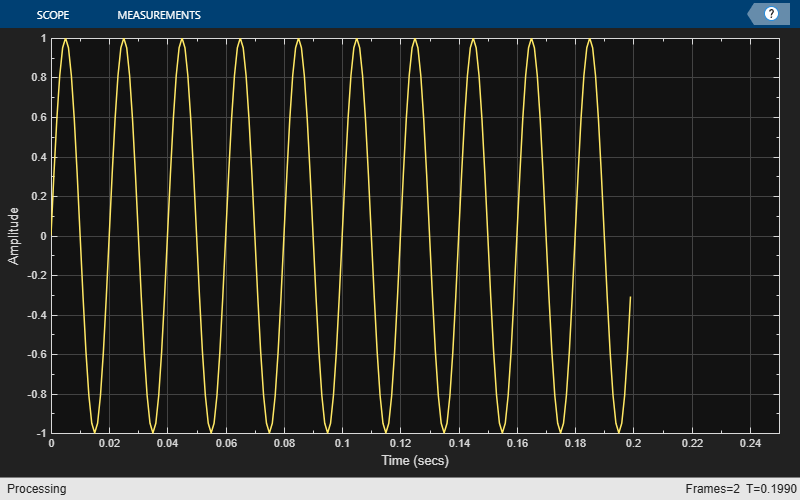# hide

Hide scope window

## Syntax

``hide(scope)``

## Description

example

````hide(scope)` hides the window of the scope.```

## Examples

collapse all

Create a sine wave signal and view it in the scope.

```Fs = 1000; % Sample rate signal = dsp.SineWave(Frequency=50,SampleRate=Fs,... SamplesPerFrame=100); scope = timescope(SampleRate=Fs,TimeSpanSource="property",... TimeSpan=0.25,YLimits=[-1 1]); for ii = 1:2 xsine = signal(); scope(xsine) end```Hide the scope window.

```if(isVisible(scope)) hide(scope) end```

Show the scope window.

```if(~isVisible(scope)) show(scope) end```Clean up workspace variables.

`clear scope Fs sine ii xsine`

## Input Arguments

collapse all

Scope object, specified as one of the following:

• `spectrumAnalyzer` object

• `dsp.ArrayPlot` object

• `dsp.LogicAnalyzer` System object™

• `timescope` object

• `dsp.DynamicFilterVisualizer` object

Example: ```myScope = timescope; hide(myScope)```

## Version History

Introduced in R2011a

expand all

Not recommended starting in R2022a